1
1
01. 云栖社区>
2. paperweekly>
3. 博客>
4. 正文

超全总结：神经网络加速之量化模型 | 附带代码

TensorLayer 是一个基于 TensorFlow 的高级开发工具，提供大量数据处理和建模 API，具备灵活性高、运行速度快等优点。今年 3 月，TensorLayer 提供了一套搭建量化网络的试验版本 API，不过目前这套 API 依然用矩阵乘法而不是加减或 bitcount 运算来加速（我们等会会提到）。

Keywords：模型压缩（Model Compression），模型加速（Model Acceleration），二值化网络（Binary Network），量化模型（Quantized Model）

DeepCompression

SongHan 这篇文章可以说是神经网络压缩领域开山之作，怎么说呢这篇文章很早就注意到了，也复现了，做了很多实验。也一直想用到硬件参数压缩以及模型加速当中，在这个过程中遇到了很多问题，现在提出来跟大家一起探讨。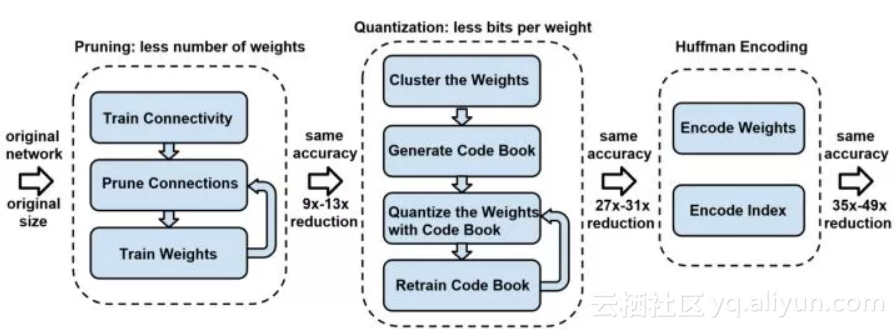DeepCompression 主要分为三个主要的部分：剪枝，量化，哈夫曼编码，下面分别探讨这几种方法并且分析他们在硬件前向配置的加速潜力。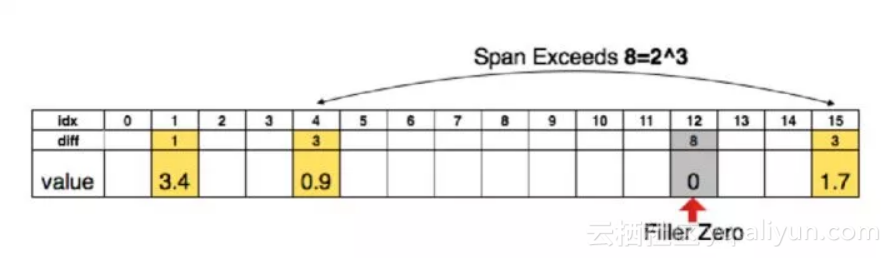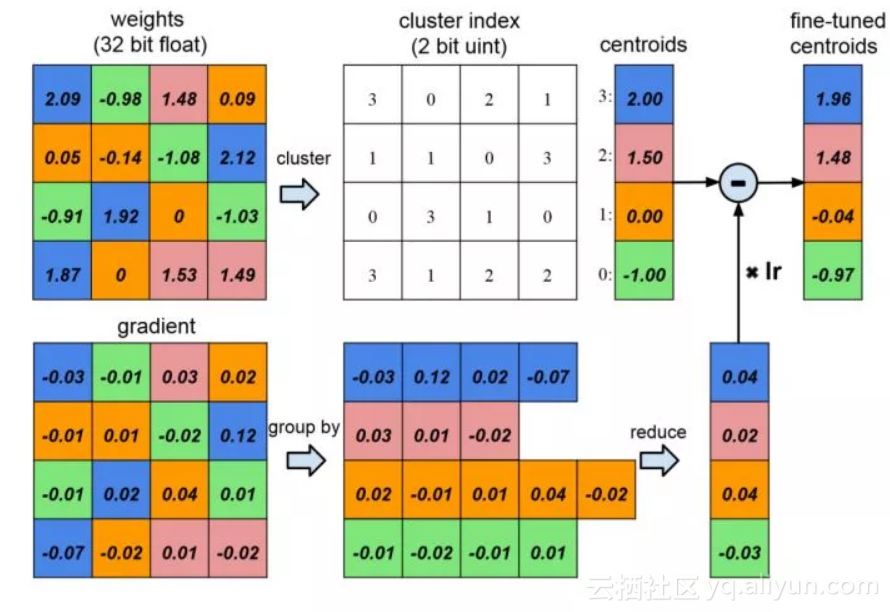Binary-Net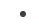Quantized Neural Networks: Training Neural Networks with Low Precision Weights and ActivationsBinarized Neural Networks: Training Deep Neural Networks with Weights and Activations Constrained to +1 or -1XNOR-Net: ImageNet Classification Using Binary Convolutional Neural Networks

1. 0 操作数的检查,即若至少有一个参与运算的数为零直接可得到结果；

2. 比较阶码大小并完成对阶；

3. 尾数进行加或减运算；

4. 结果规格化并进行舍入处理。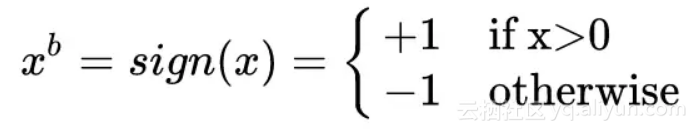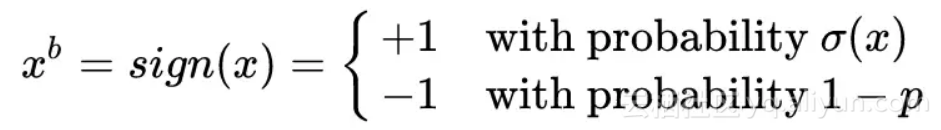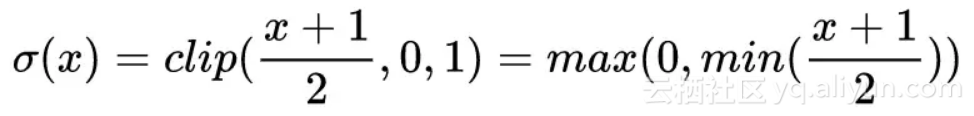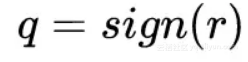C 对 q 的的导数使用 gq 表示，那么 q 对 r 的导数就变成了：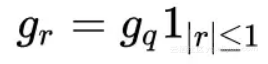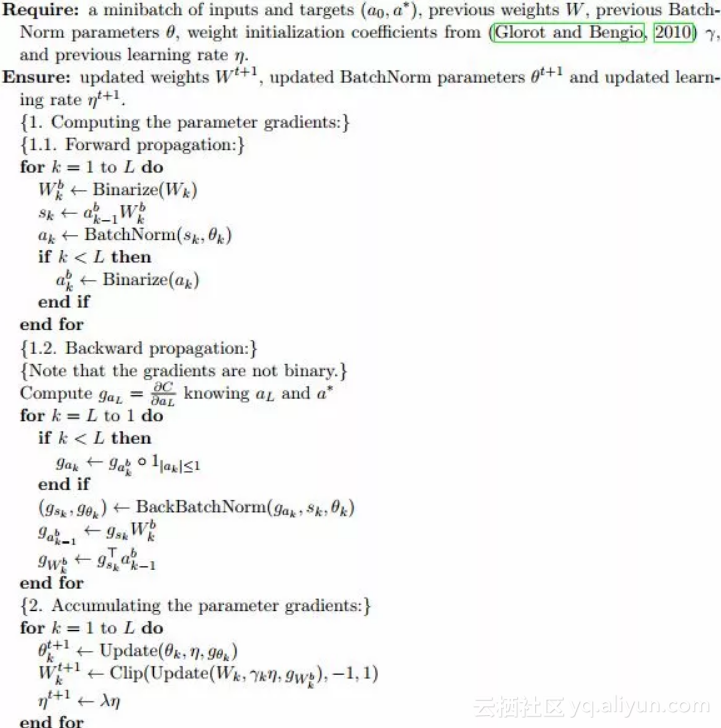BN 最大的作用就是加速学习，减少权重尺度影响，带来一定量的正则化，可以提高网络性能，但是，BN 涉及很多矩阵运算（matrix multiplication），会降低运算速度，因此，提出了一种 shift-based Batch Normalization。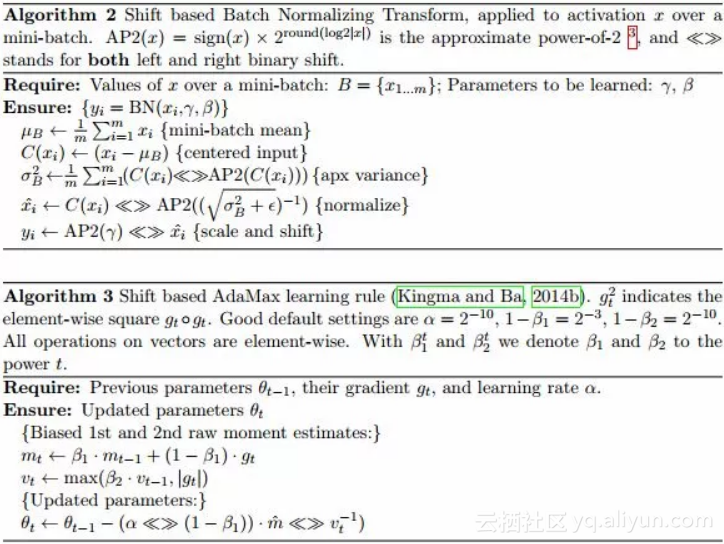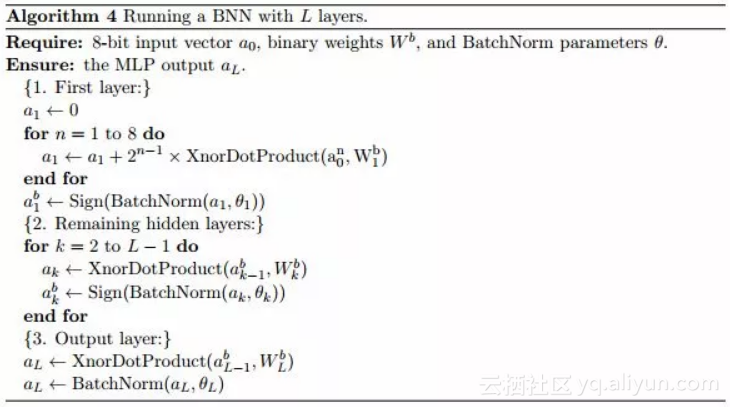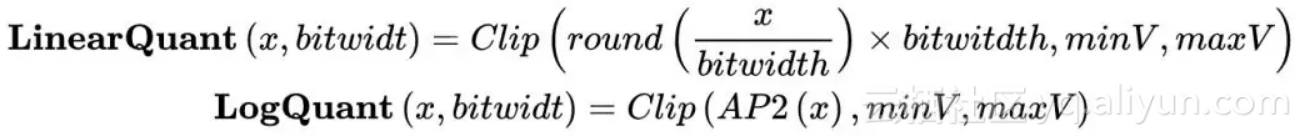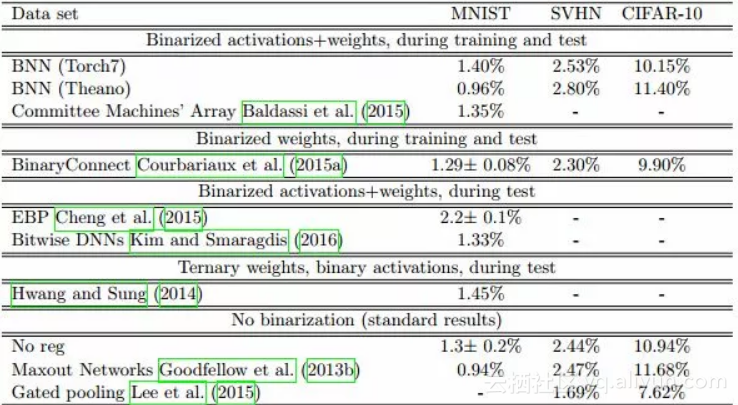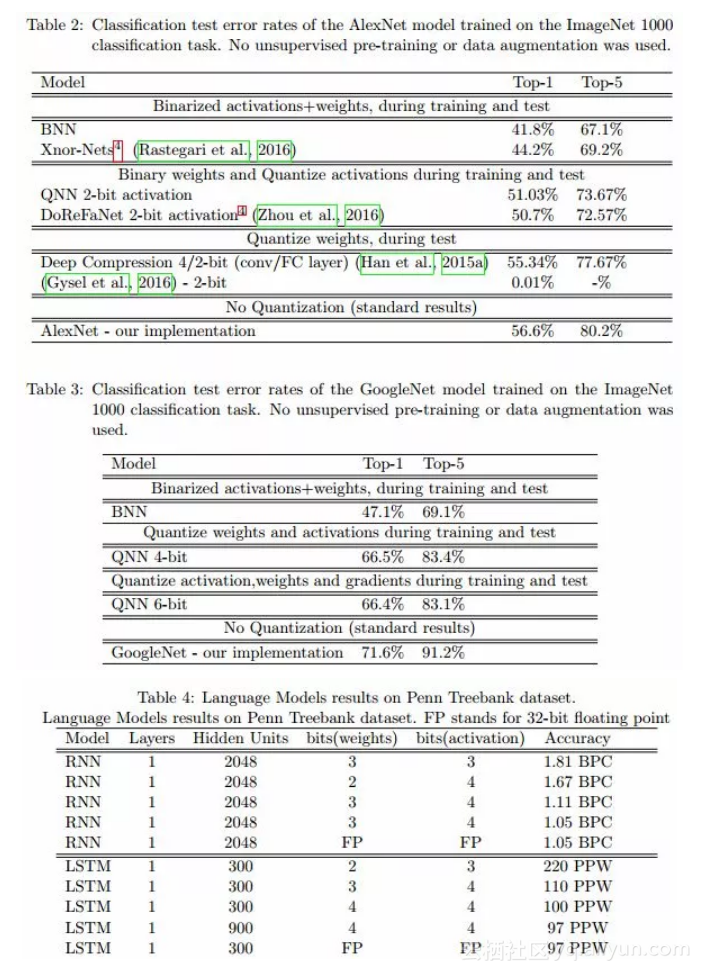x = tf.placeholder(tf.float32, shape=[batch_size, 28, 28, 1])
net = tl.layers.InputLayer(x, name='input')
net = tl.layers.BinaryConv2d(net, 32, (5, 5), (1, 1), padding='SAME', b_init=None, name='bcnn1')
net = tl.layers.MaxPool2d(net, (2, 2), (2, 2), padding='SAME', name='pool1')
net = tl.layers.BatchNormLayer(net, act=tl.act.htanh, is_train=is_train, name='bn1')

net = tl.layers.SignLayer(net)
net = tl.layers.BinaryConv2d(net, 64, (5, 5), (1, 1), padding='SAME', b_init=None, name='bcnn2')
net = tl.layers.MaxPool2d(net, (2, 2), (2, 2), padding='SAME', name='pool2')
net = tl.layers.BatchNormLayer(net, act=tl.act.htanh, is_train=is_train, name='bn2')

net = tl.layers.FlattenLayer(net)

net = tl.layers.SignLayer(net)
net = tl.layers.BinaryDenseLayer(net, 256, b_init=None, name='dense')
net = tl.layers.BatchNormLayer(net, act=tl.act.htanh, is_train=is_train, name='bn3')

net = tl.layers.SignLayer(net)
net = tl.layers.BinaryDenseLayer(net, 10, b_init=None, name='bout')
net = tl.layers.BatchNormLayer(net, is_train=is_train, name='bno')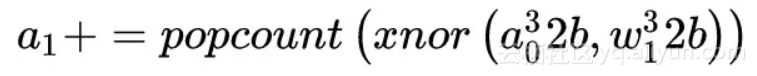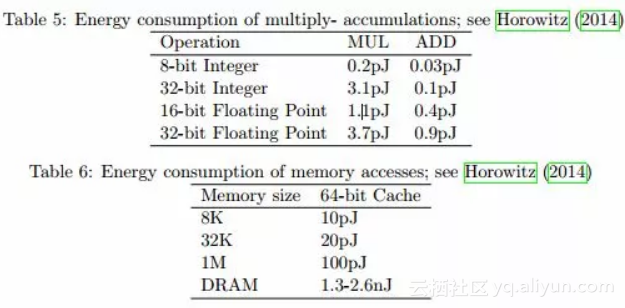Xnor-Net 在 BNN 的基础上引入了比例因子，让二值化之后的参数和原始的参数的 L2 范数最小，提高了模型的精度。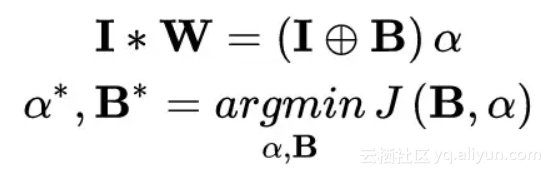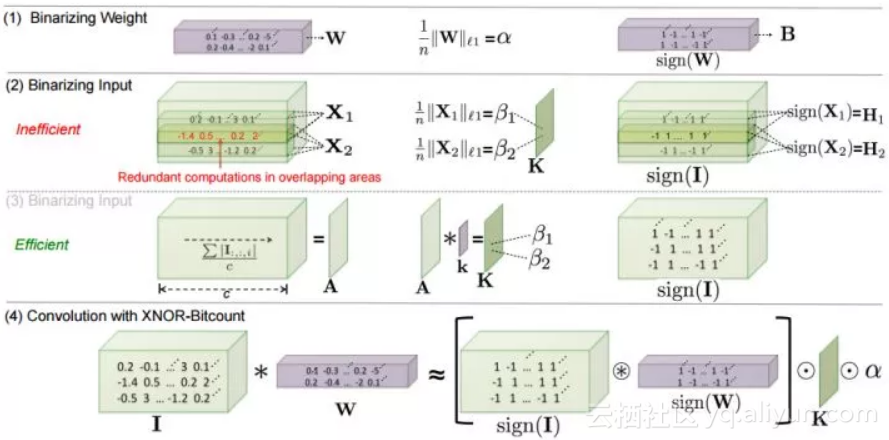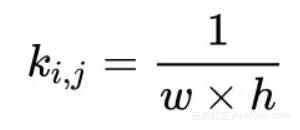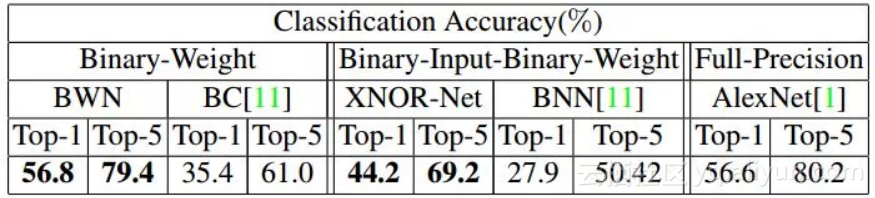Ternary-NetTernary Weight Networks paper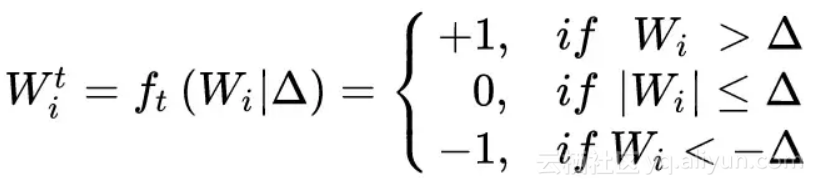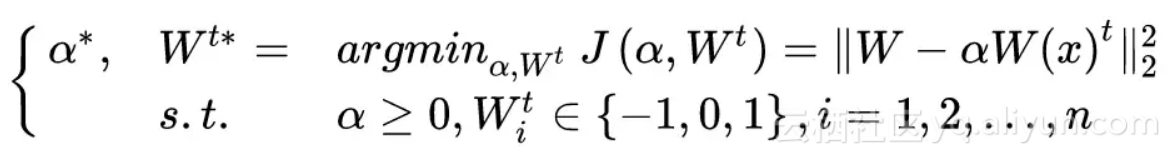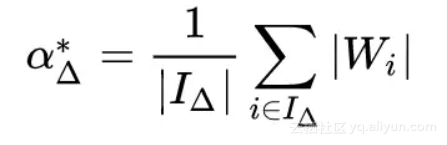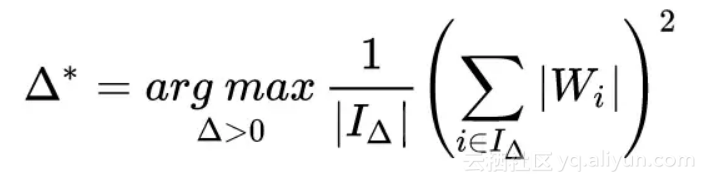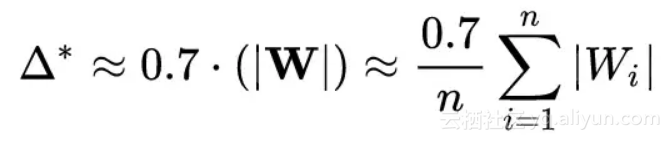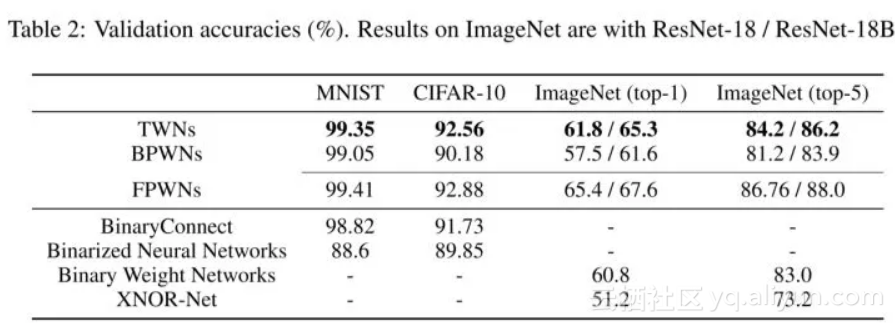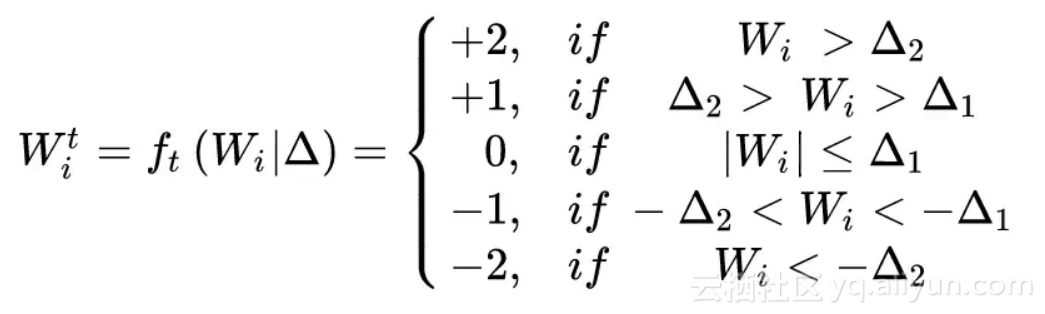Scale 参数的计算公式变成了：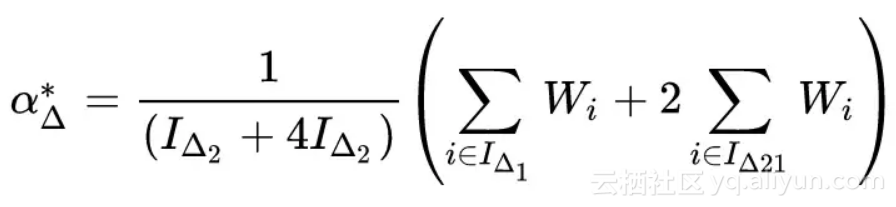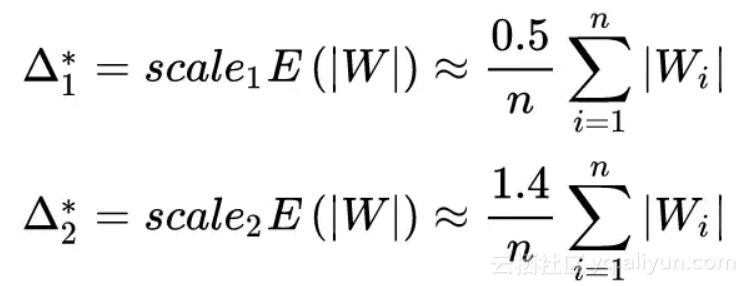net = tl.layers.InputLayer(x, name='input')
net = tl.layers.TernaryConv2d(net, 32, (5, 5), (1, 1), padding='SAME', b_init=None, name='bcnn1')
net = tl.layers.MaxPool2d(net, (2, 2), (2, 2), padding='SAME', name='pool1')
net = tl.layers.BatchNormLayer(net, act=tl.act.htanh, is_train=is_train, name='bn1')
net = tl.layers.TernaryConv2d(net, 64, (5, 5), (1, 1), padding='SAME', b_init=None, name='bcnn2')
net = tl.layers.MaxPool2d(net, (2, 2), (2, 2), padding='SAME', name='pool2')
net = tl.layers.BatchNormLayer(net, act=tl.act.htanh, is_train=is_train, name='bn2')

net = tl.layers.FlattenLayer(net)
net = tl.layers.TernaryDenseLayer(net, 256, b_init=None, name='dense')
net = tl.layers.BatchNormLayer(net, act=tl.act.htanh, is_train=is_train, name='bn3')
net = tl.layers.TernaryDenseLayer(net, 10, b_init=None, name='bout')
net = tl.layers.BatchNormLayer(net, is_train=is_train, name='bno')
return net

DoReFa-NetDoReFa-Net: Training Low Bitwidth Convolutional Neural Networks with Low Bitwidth Gradients

Face++ 团队在 16 年 6 月提出的 Dorefa-Net 和上面两种量化方法思路也是比较接近，但 DoReLa-Net 对比例因子的设计更为简单，这里并没有针对卷积层输出的每一个过滤映射计算比例因子，而是对卷积层的整体输出计算一个均值常量作为比例因子。这样的做法可以简化反向运算，因为在他们反向计算时也要实现量化。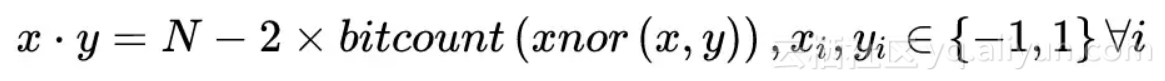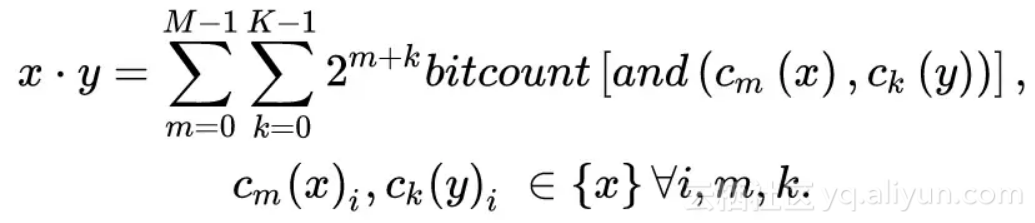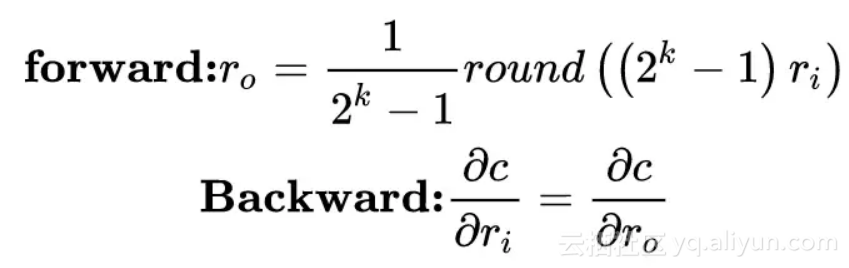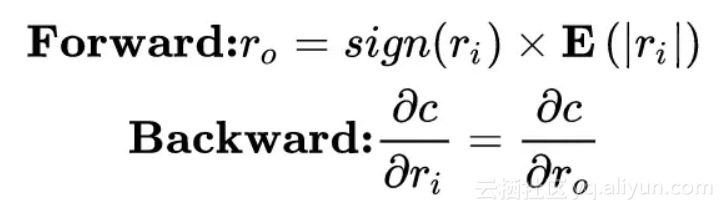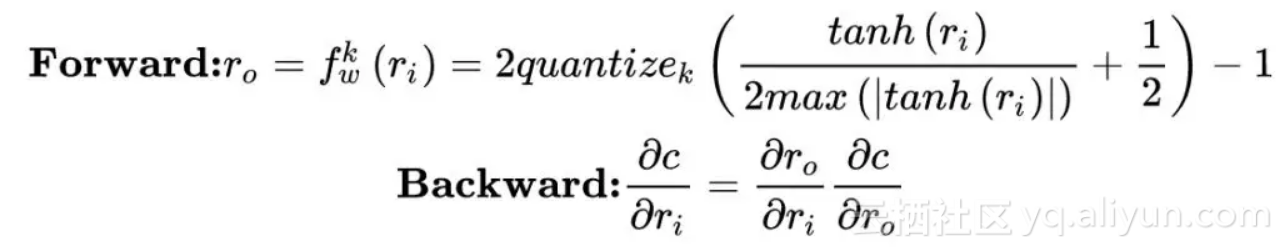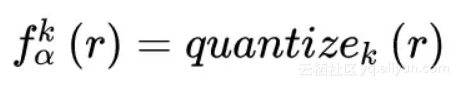DoReFa 的梯度量化方法比较复杂，因为梯度是无界的，并且可能具有比隐层输出更大的值范围。我们可以通过使可微分非线性函数传递值来将隐层输出范围映射到 [0,1]。 但是，这种构造不适用于渐变。 文章设计了以下用于梯度 k 位量化的函数，这里 dr 是 r 对损失函数 C 的偏导。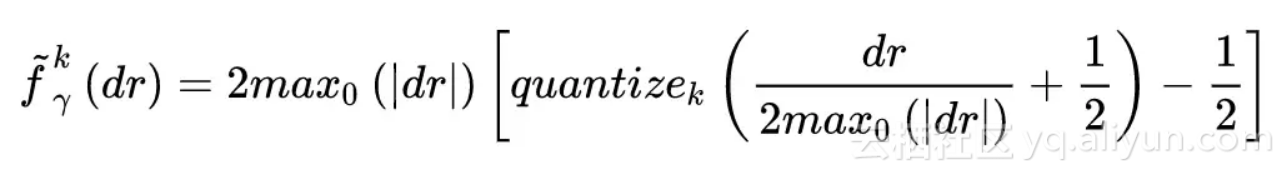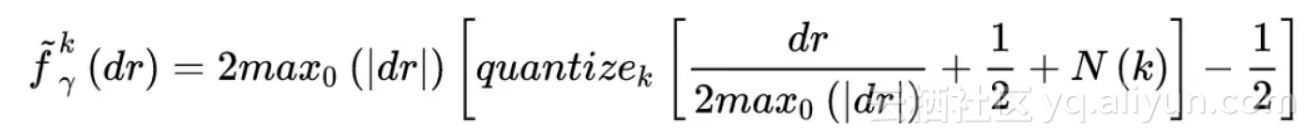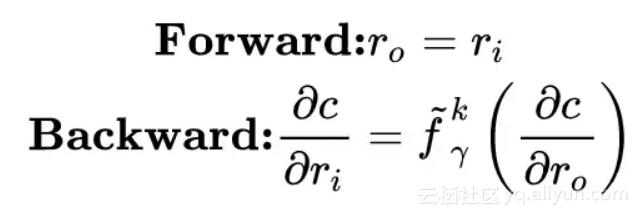DoReFa-net 为了进一步节省资源将 3,4,6 步放在一起做，将 11,12 步融合在一起，节省了中间步骤的全精度数储存消耗的资源。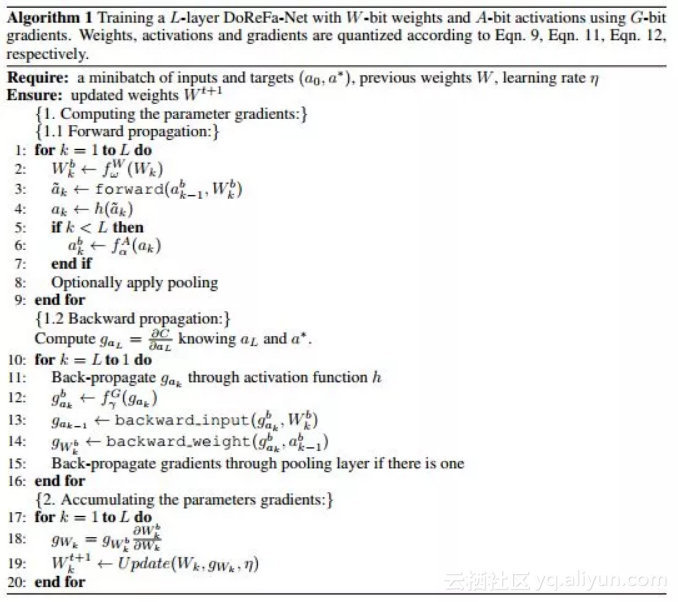DoReFa-Net 分别对 SVHN 和 ImageNet 进行了实验，准确率如下：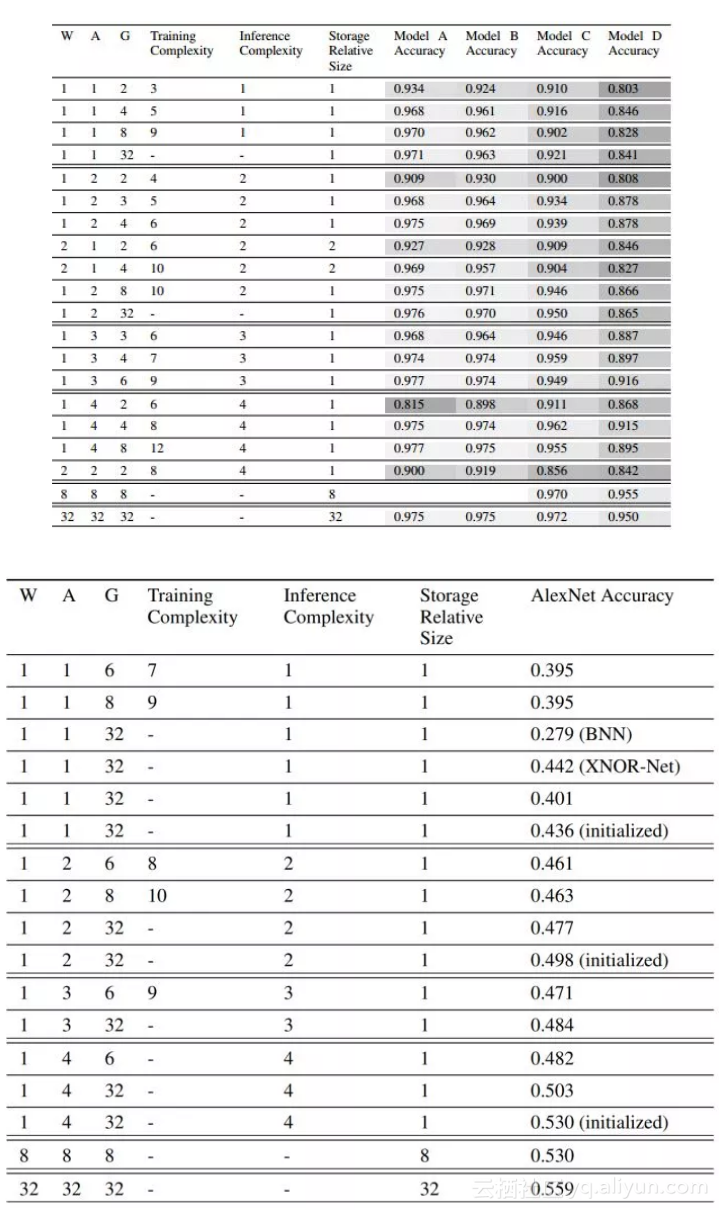net = tl.layers.InputLayer(x, name='input')
net = tl.layers.DorefaConv2d(net, 1, 3, 32, (5, 5), (1, 1), padding='SAME', b_init=None, name='bcnn1') #pylint: disable=bare-except
net = tl.layers.MaxPool2d(net, (2, 2), (2, 2), padding='SAME', name='pool1')
net = tl.layers.BatchNormLayer(net, act=tl.act.htanh, is_train=is_train, name='bn1')
net = tl.layers.DorefaConv2d(net, 1, 3, 64, (5, 5), (1, 1), padding='SAME', b_init=None, name='bcnn2') #pylint: disable=bare-except
net = tl.layers.MaxPool2d(net, (2, 2), (2, 2), padding='SAME', name='pool2')
net = tl.layers.BatchNormLayer(net, act=tl.act.htanh, is_train=is_train, name='bn2')

net = tl.layers.FlattenLayer(net)
net = tl.layers.DorefaDenseLayer(net, 1, 3, 256, b_init=None, name='dense')
net = tl.layers.BatchNormLayer(net, act=tl.act.htanh, is_train=is_train, name='bn3')
net = tl.layers.DenseLayer(net, 10, b_init=None, name='bout')
net = tl.layers.BatchNormLayer(net, is_train=is_train, name='bno')A Survey of Model Compression and Acceleration for Deep Neural Networks (end of 2017)Channel Pruning for Accelerating Very Deep Neural NetworksSqueezeNet: AlexNet-level accuracy with 50x fewer parameters and <0.5MB model sizeMobileNets: Efficient Convolutional Neural Networks for Mobile Vision ApplicationsShuffleNet: An Extremely Efficient Convolutional Neural Network for Mobile Devices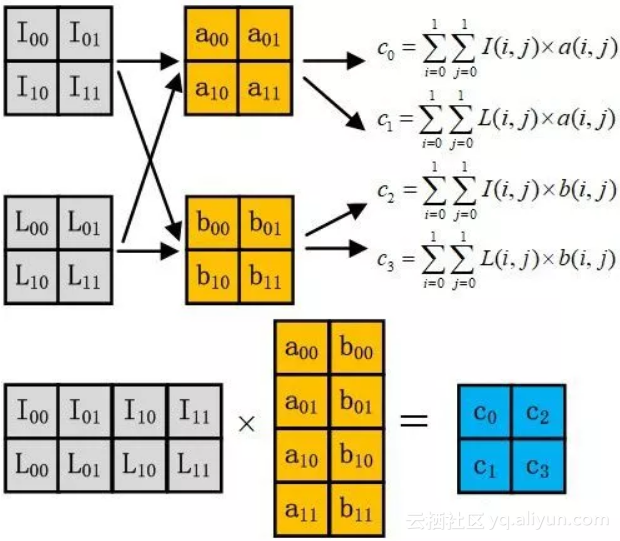GPU优化：

1. 了解 IO 访问的情况以及 IO 的性能；

2. 多线程的并行计算特性；

3. IO 和并行计算间的计算时间重叠。

Strassen 算法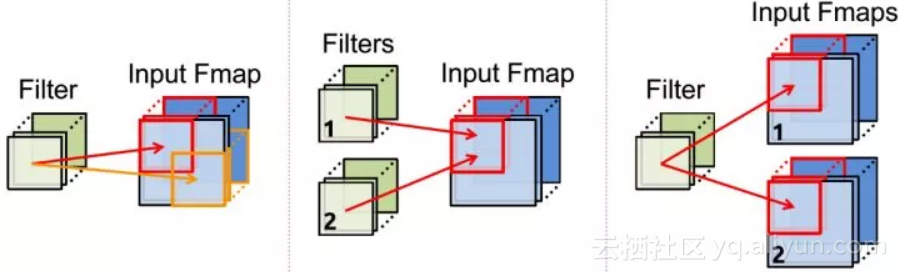NLR (No Local Reuse)：使用大型全局缓冲区共享存储，减少 DRAM 访问能耗；

RS：在内部的寄存器中最大化重用和累加，针对整体能源效率进行优化，而不是只针对某种数据类型。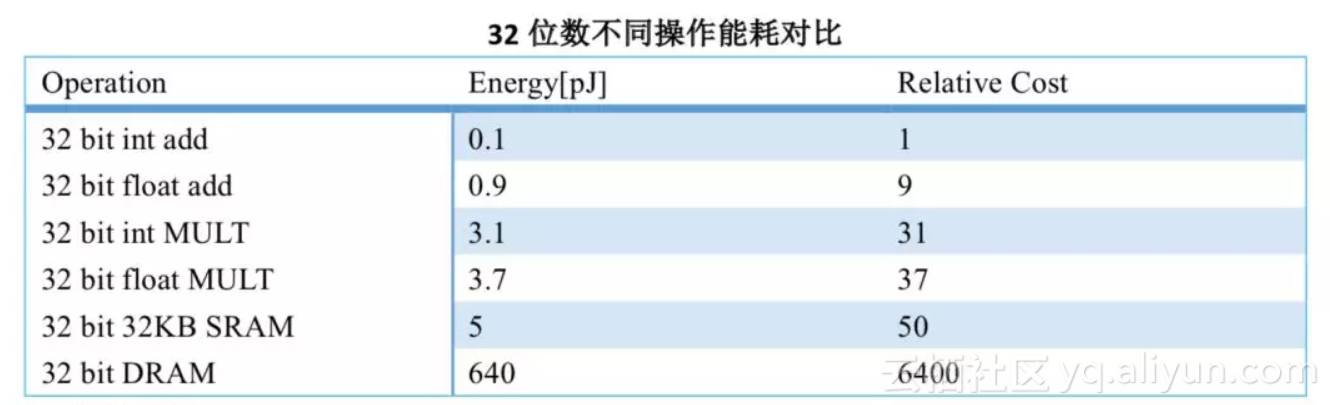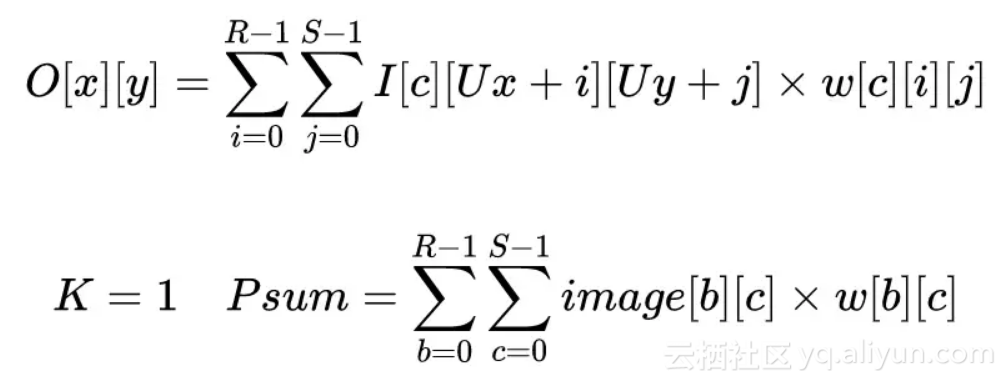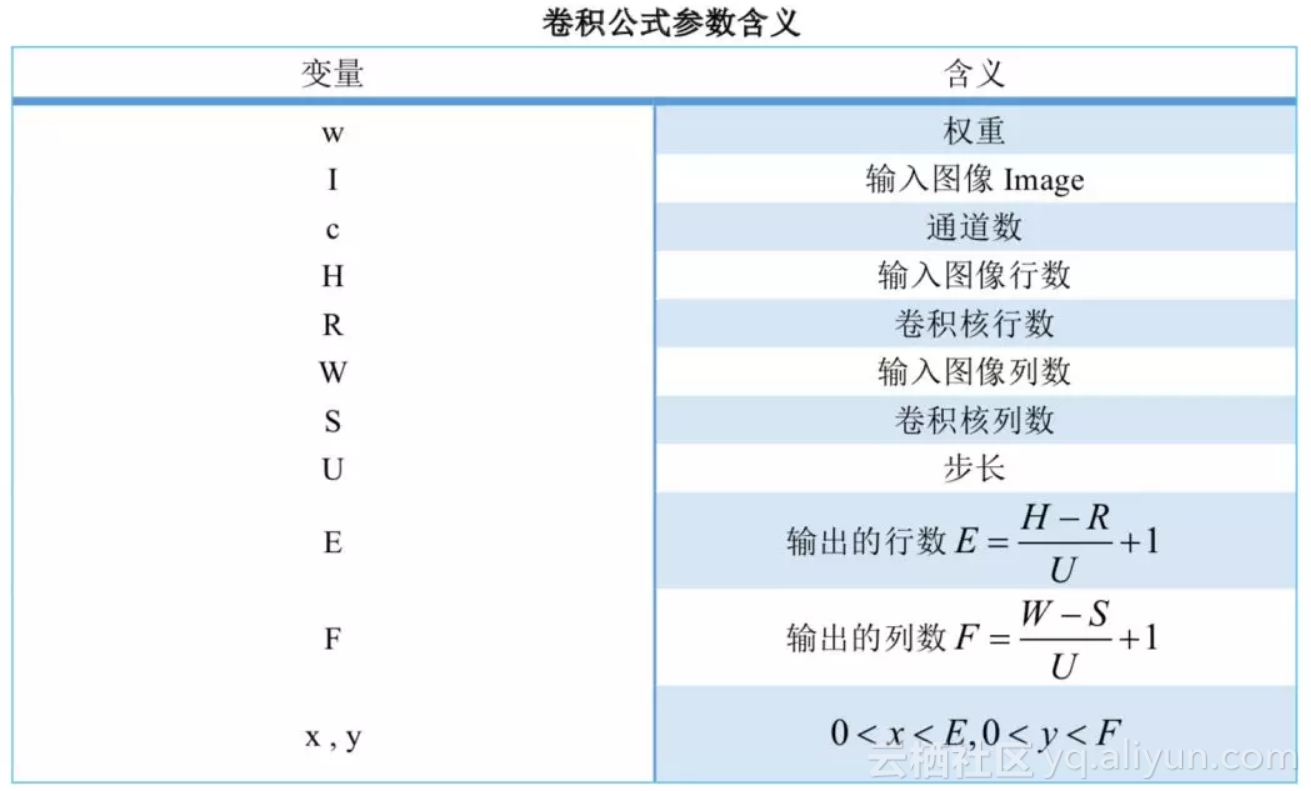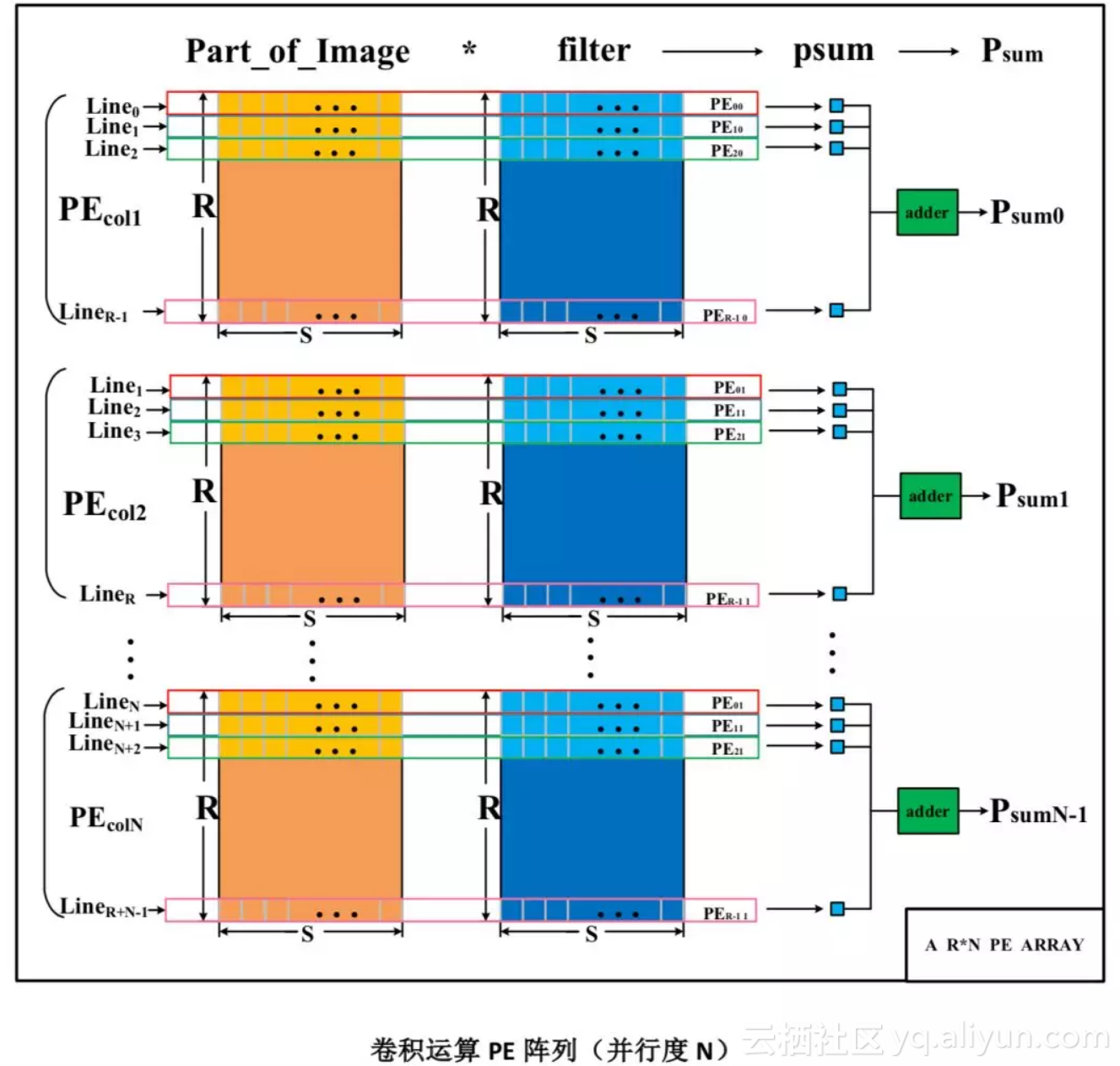+ 关注